# In a market with a duopoly, if market demand is find the Cournot Reaction curves and...

In a market with a duopoly, if market demand is find the Cournot Reaction curves and the Cournot quantity solutions then deduce the price in the case where Marginal cost curves for either of the duopoly firms is and . Compare your results to the case where a Monopolist that has a replaces the duopoly. What are the monopoly quantity and price? Which quantities are bigger, Cournot or Monopoly? What is the consumer Surplus in both cases? Set up the oligopoly model in a game-theoretical prisoner's dilemma framework. Explain briefly the strategies and how you reach the Nash Equilibrium.

P = 300 - Q
Mc = 91 +30
Mc2 = 42 +30
MC=Q +30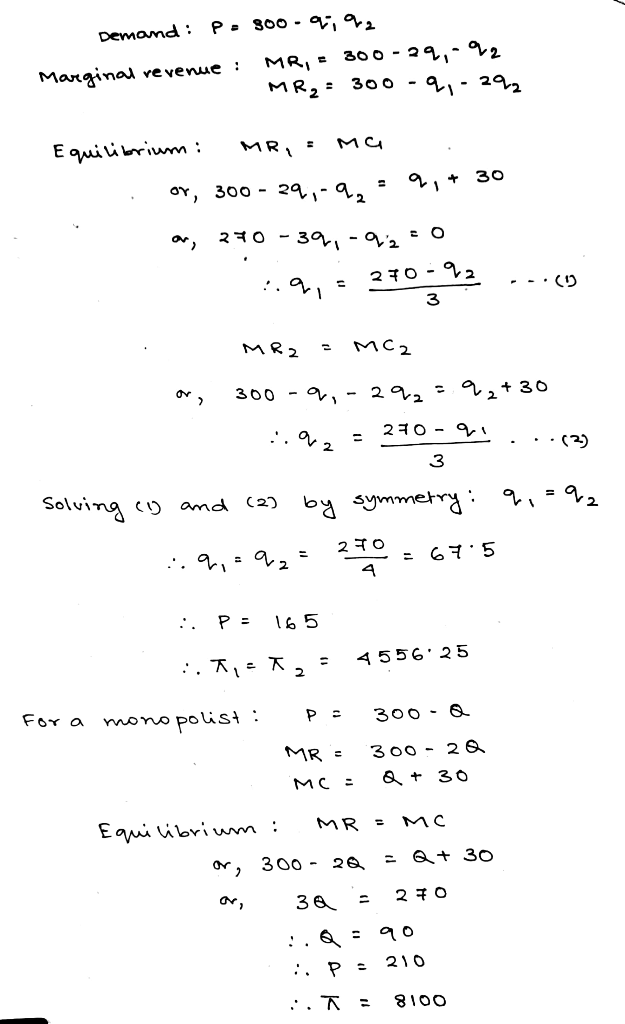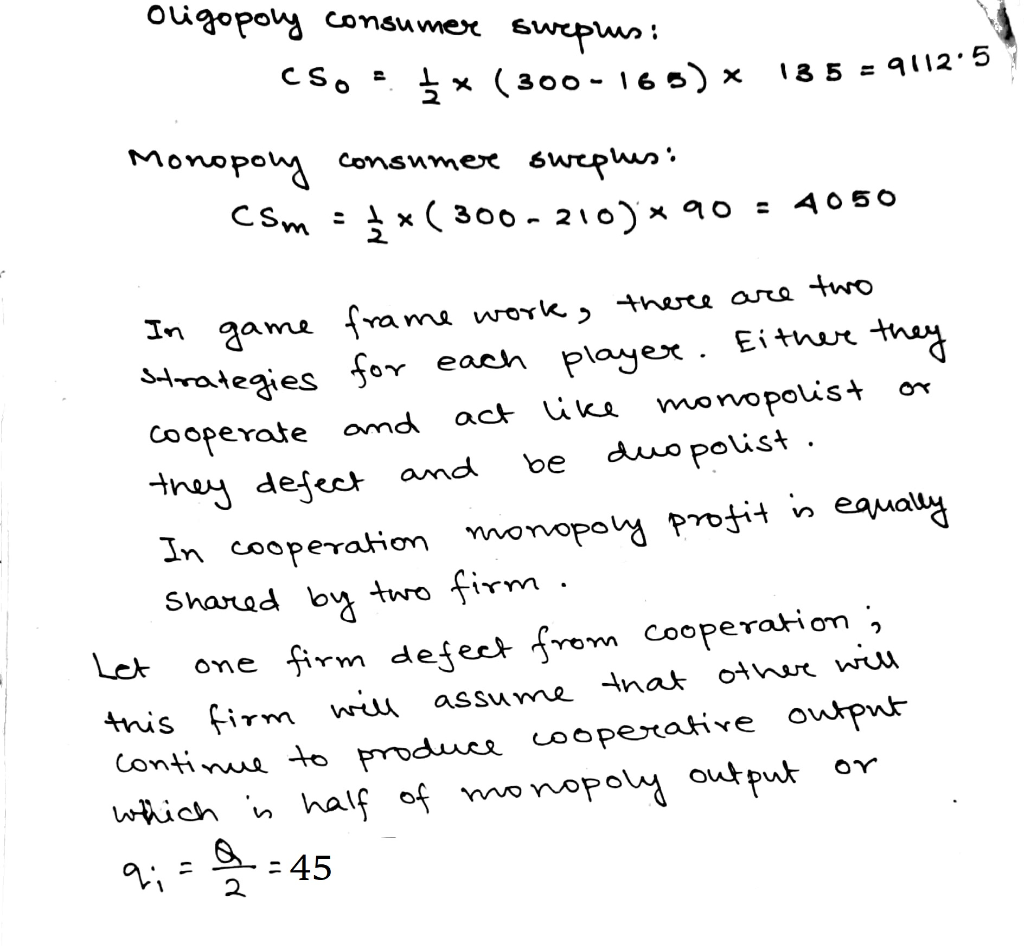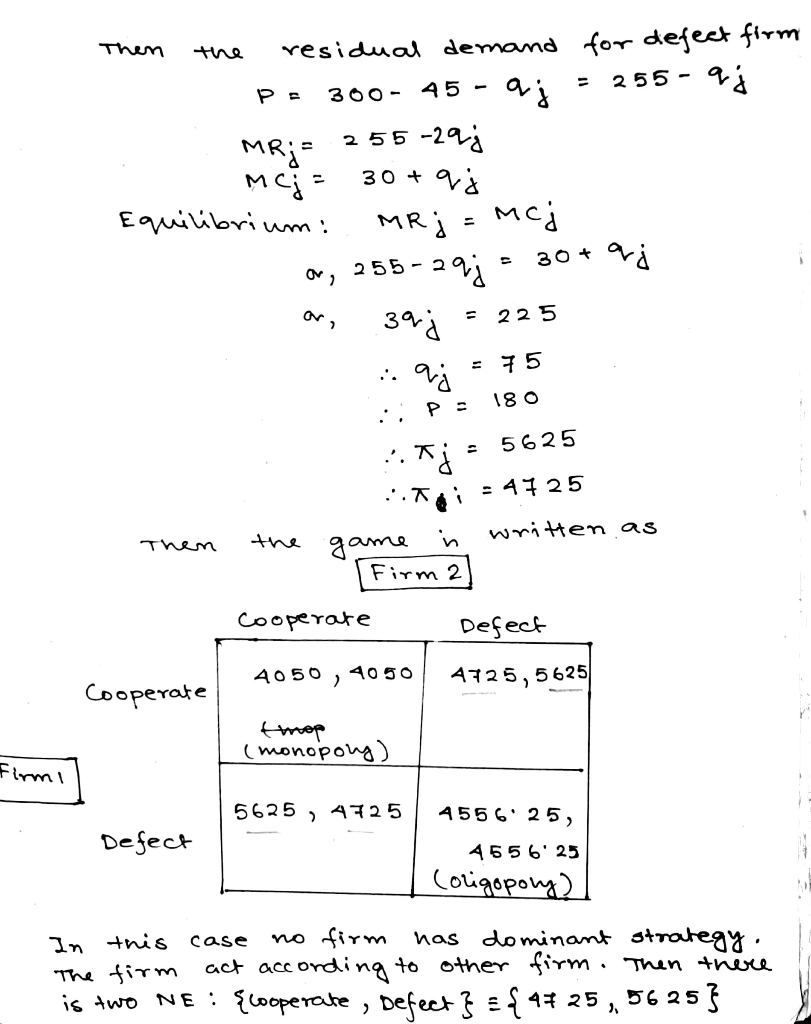##### Add Answer of: In a market with a duopoly, if market demand is find the Cournot Reaction curves and...
Similar Homework Help Questions
• ### Problem 2. Gibbons 1.5 Consider the following two finite versions of the Cournot duopoly model. First,...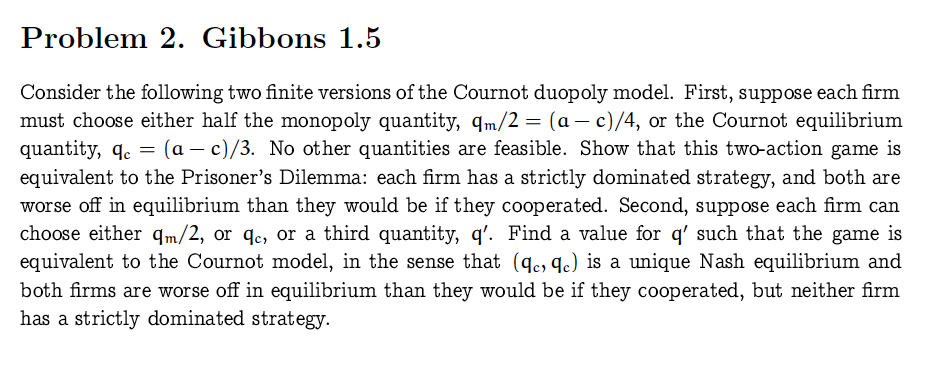Problem 2. Gibbons 1.5 Consider the following two finite versions of the Cournot duopoly model. First, suppose each firm must choose either half the monopoly quantity, 4m/2 = (a - c)/4, or the Cournot equilibrium quantity, 4c = (a - c)/3. No other quantities are feasible. Show that this two-action game is equivalent to the Prisoner's Dilemma: each firm has a strictly dominated strategy, and both are worse off in equilibrium than they would be if they cooperated. Second, suppose...

• ### A duopoly faces a market demand of p 180-Q. Firm 1 has a constant marginal cost...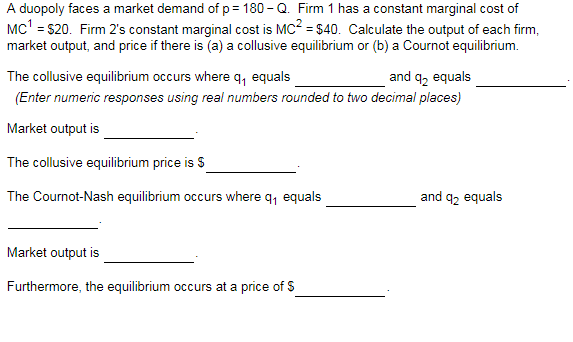A duopoly faces a market demand of p 180-Q. Firm 1 has a constant marginal cost of Mc1 -S20. Firm 2s constant marginal cost is MC2 \$40. Calculate the output of each firm, market output, and price if there is (a) a collusive equilibrium or (b) a Cournot equilibrium The collusive equilibrium occurs where q, equals and q2 equals (Enter numeric responses using real numbers rounded to two decimal places) Market output is The collusive equilibrium price is S The...

• ### (16 points) Cournot Duopoly. Market demand is p(Q) = 50 – 4Q, where Q = 4+...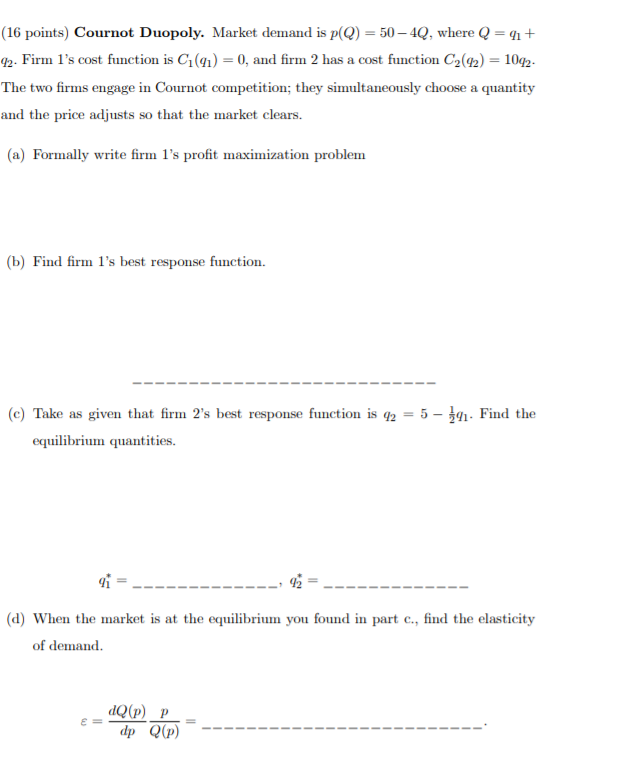(16 points) Cournot Duopoly. Market demand is p(Q) = 50 – 4Q, where Q = 4+ 42. Firm 1's cost function is C (91) = 0, and firm 2 has a cost function C2(92) = 1092- The two firms engage in Cournot competition; they simultaneously choose a quantity and the price adjusts so that the market clears. (a) Formally write firm 1's profit maximization problem (b) Find firm l's best response function. (c) Take as given that firm 2's best...

• ### 2. (Cournot Model) Consider a Cournot duopoly. The market demand is p=160 - q2. Firm 1's...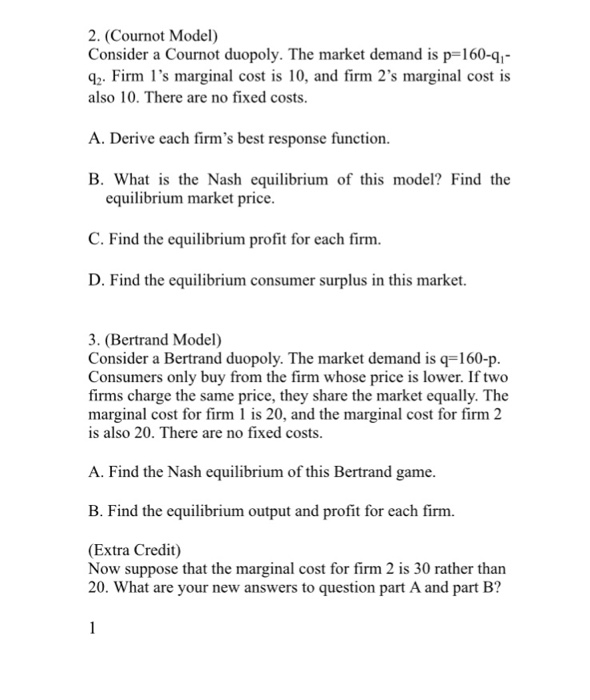2. (Cournot Model) Consider a Cournot duopoly. The market demand is p=160 - q2. Firm 1's marginal cost is 10, and firm 2's marginal cost is also 10. There are no fixed costs. A. Derive each firm's best response function B. What is the Nash equilibrium of this model? Find the equilibrium market price. C. Find the equilibrium profit for each firm D. Find the equilibrium consumer surplus in this market. 3. (Bertrand Model) Consider a Bertrand duopoly. The market...

• ### Suppose that the market demand curve for mineral water is given as Q=100−10P and marginal cost...

Suppose that the market demand curve for mineral water is given as Q=100−10P and marginal cost is fixed at \$4. Find the equilibrium price and quantity in each type of different market structure. Show your calculation. a) Monopoly b) Cournot duopoly c) Stackelberg duopoly d) Bertrand duopoly (MR is fixed at the level of MC). e) Perfect competitive market (MR is fixed at the level of MC).

• ### A homogeneous product duopoly faces a market demand function given by p = 300 - 3Q,where...

A homogeneous product duopoly faces a market demand function given by p = 300 - 3Q,where Q = q1 + q2. Both firms have constant marginal cost MC = 100. (part 2) 1a. What is the Bertrand equilibrium price and quantity in this market? 1b. Suppose Firm 1 is the Stackelberg leader, what is the equilibrium price in this market if Firm 2 plays the follower in this duopoly market? What is the equilibrium quantity? How much does each firm...

• ### 7. Consider an asymmetric Cournot duopoly game, where the two firms have different costs of production....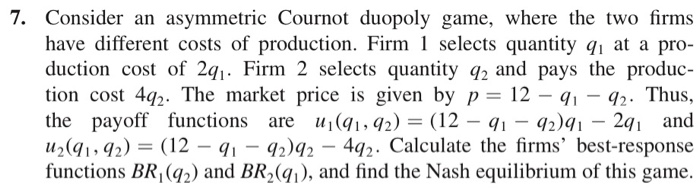7. Consider an asymmetric Cournot duopoly game, where the two firms have different costs of production. Firm 1 selects quantity qı at a pro- duction cost of 291. Firm 2 selects quantity 92 and pays the produc- tion cost 492. The market price is given by p = 12 – 91 - 92. Thus, the payoff functions are u(91,92) = (12 – 91 - 92.91 – 291 and uz(9192) = (12 – 91 - 92)92 – 492. Calculate the firms'...

• ### Suppose that the market demand curve for mineral water is given as ?=100−10? and marginal cost...

Suppose that the market demand curve for mineral water is given as ?=100−10? and marginal cost is fixed at \$4. Find the equilibrium price and quantity in each type of different market structure. Show your calculation.   a) Monopoly, Cournot duopoly, and Stackelberg duopoly b)Bertrand duopoly (MR is fixed at the level of MC). c) Perfect competitive market (MR is fixed at the level of MC).

• ### Suppose that the market demand curve for mineral water is given as ?=100−10? and marginal cost...

Suppose that the market demand curve for mineral water is given as ?=100−10? and marginal cost is fixed at \$4. Find the equilibrium price and quantity in each type of different market structure. Show your calculation. 1) Monopoly, Cournot duopoly, and Stackelberg duopoly 2)Bertrand duopoly (MR is fixed at the level of MC). 3) Perfect competitive market (MR is fixed at the level of MC).

• ### 2) (30 pts) Suppose that the market demand for soft drinks in a small town in...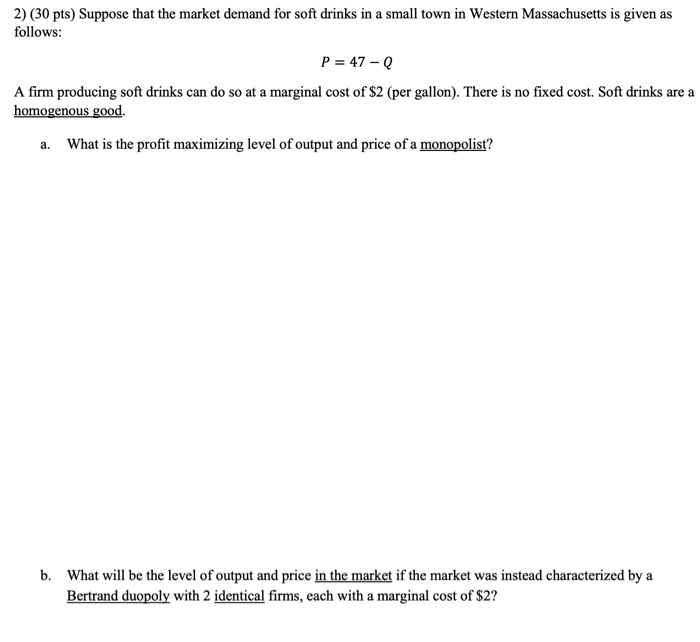2) (30 pts) Suppose that the market demand for soft drinks in a small town in Western Massachusetts is given as follows: P = 47-Q A firm producing soft drinks can do so at a marginal cost of \$2 (per gallon). There is no fixed cost. Soft drinks are a homogenous good. a. What is the profit maximizing level of output and price of a monopolist? b. What will be the level of output and price in the market if...

Free Homework App